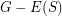# Cycle Double Covers Containing Predefined 2-Regular Subgraphs

 Importance: High ✭✭✭
 Author(s): Arthur Hoffmann-Ostenhof
 Subject: Graph Theory
 Keywords:
Conjecture   Letbe a-connected cubic graph and letbe a-regular subgraph such thatis connected. Thenhas a cycle double cover which contains(i.e all cycles of).
Used definitions in the above conjecture: a "cycle" is a connected 2-regular subgraph, a "cycle double cover" of a graphis a set of cycles ofsuch that every edge ofis contained in precisely two cycles of the set. This conjecture has been motivated by Theorem 3, respectively, Theorem 4 in www.arxiv.org/abs/1711.10614. A weaker conjecture (Conjecture 14) has been stated in "Snarks with special spanning trees" (see www.arxiv.org/abs/1706.05595).